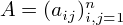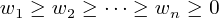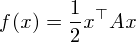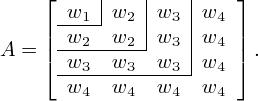DCP HOME

# Quadratic M-Convex Minimization

For a symmetric matrixwithfor some, the associated quadratic formis an M-convex function [1,2]. For n=4, such a matrix looks likeHere we consider a quadratic M-convex function:.

In this web application, you can choose the dimension n from 1 to 7.

dimension n =

You can input parameters aij and bi in the ranges 0 < aij≤ 50 and -50 ≤ bi≤ 50. If the input does not satisfy the condition, the unsatisfactory condition turns red.

You can also input an initial solution x.

 A 1 2 3 1 2 3

 1 2 3 b x

(Note: "Reset" button resets your inputs to default values.)

This web application minimizes f(x) using ODICON.

 K. Murota (2001): "Discrete Convex Analysis---An Introduction (in Japanese)," Kyoritsu Publishing Company, Tokyo. Section 5.6.

 K. Murota (2003): "Discrete Convex Analysis," SIAM. Section 6.3.